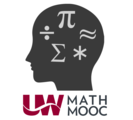#Math MOOC Summer 2019 is a Course

## Math MOOC Summer 2019

Ended Sep 30, 2019
1 credit

### Full course description

The College Readiness Math MOOC (Massive Open Online Course) is a self-paced online program designed to enhance your mathematics skills in the areas of algebra and geometry. This MOOC is designed to help develop the skills needed to be successful in college mathematics.

The Math MOOC is comprised of ten math modules that have been aligned to specific learning objectives topically found in a developmental mathematics course. These learning objectives include:
Course Objectives: Upon completion of this course, students will be able to:
Module 1: Fractions, Ratios, and Percents
·       Perform operations with real numbers
·       Use rates, ratios, and percents to solve real-world and mathematical problems
Module 2: Geometry and Measurement
·       Use measurement formulas to model and solve geometric problems
·       Solve problems involving special triangles including the relationship between sides and angles of these special triangles
·       Apply the Pythagorean’s Theorem to solve real-world and mathematical problems related to right triangles
·       Solve problems involving geometric figures using the distance and midpoint formulas
Module 3:  Solving Linear Equations, Inequalities, and Absolute Value
·       Create and solve linear equations and inequalities in one variable related to real-world and mathematical problems
·       Solve equations and inequalities involving the absolute value
Module 4: Graphing Linear Equations
·       Graph linear equations in two variables
·       Construct the equation of a line in point-slope and slope-intercept form to solve real-world and mathematical problems
·       Determine the relationship between the slopes of parallel and perpendicular lines
Module 5: Systems of Linear Equations
·       Solve systems of equations algebraically and graphically related to real-world and mathematical problems
·       Apply the laws of exponents to simplify expressions involving to rational exponents and solve problems involving scientific notation
·       Apply the properties of radicals to simplify expressions involving square roots
Module 7: Polynomial Operations
·       Perform operations with polynomial expressions
·       Identify a relationship between two quantities that satisfies the definition of a function
·       Use function notation to analyze mathematical situations
Module 8: Factoring
·       Factor polynomial expressions including quadratic expressions